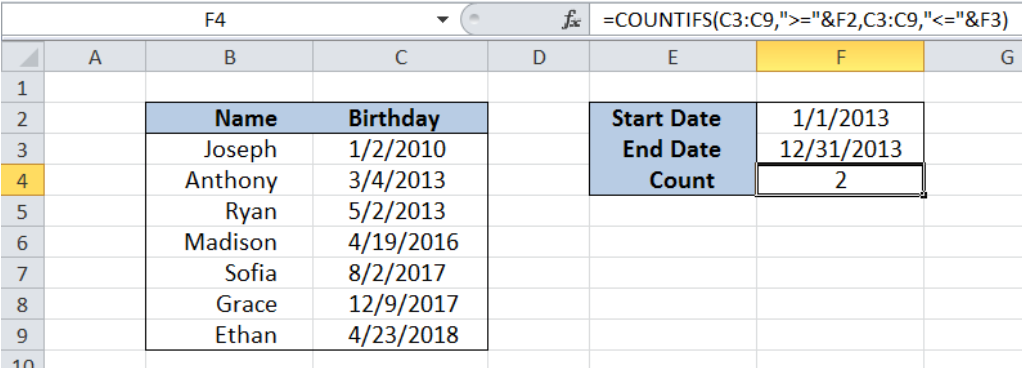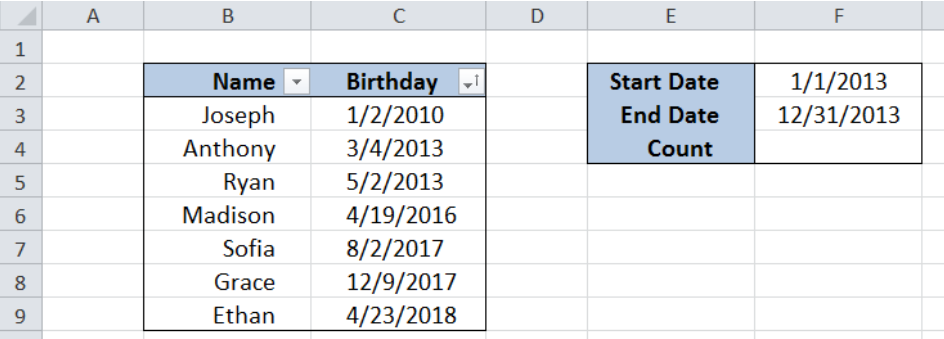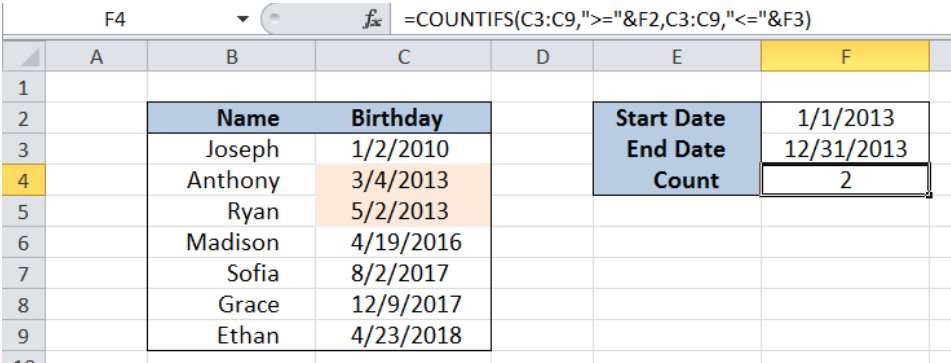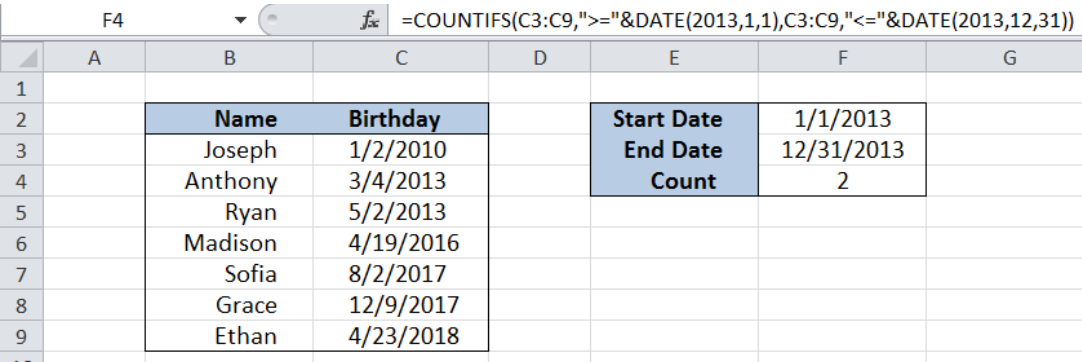Get instant live expert help with Excel or Google Sheets“My Excelchat expert helped me in less than 20 minutes, saving me what would have been 5 hours of work!”

#### Post your problem and you’ll get Expert help in seconds.

Your message must be at least 40 characters
Our professional Expert are available now. Your privacy is guaranteed.

# Count cells between dates

Excel allows us to count cells between dates by using the COUNTIFS function. The COUNTIFS function counts the number of cells that meet one or more criteria.  This step by step tutorial will assist all levels of Excel users in counting cells between dates.Figure 1. Final result: Count cells between dates

Final formula: `=COUNTIFS(C3:C9,">="&F2,C3:C9,"<="&F3)`

## Syntax of COUNTIFS Function

`=COUNTIFS(criteria_range1, criteria1, [criteria_range2, criteria2]…)`

Parameters:

• Criteria_range1: the data range that will be evaluated using the criteria1
• Criteria1:  the criteria or condition that determines which cells will be counted
• Criteria_range2 and criteria2 are optional; only applied when there are more than one criteria as specified

## Setting up Our Data

Our table contains a list of names (column B) and birthdays (column C).  Cells F2 and F3 contain the Start Date and End Date, which will be our criteria in counting the number of cells in between these dates.  The resulting count will be in cell F4.Figure 2. Sample data to count cells between dates

## Count Cells Between Dates using Cell Reference

We want to determine the number of celebrants whose birthdays fall on 2013, where the start date is January 1, 2013 (1/1/2013) , while the end date is December 31, 2013 (12/31/2013).

To count the number of cells between the specified dates, we will follow these steps:

Step 1.  Select cell F4

Step 2.  Enter the formula: `=COUNTIFS(C3:C9,">="&F2,C3:C9,"<="&F3)`

Step 3: Press ENTER

Our formula has two criteria.  It counts the dates that fall on or after 1/1/2013, and at the same time fall on or before 12/31/2013.  The symbol “>=” means “on or after” while “<=” means “on or before”.  The ampersand “&” is used to link the symbols and the dates specified in cells F2 and F3.Figure 3. Using the COUNTIFS function to count cells between dates

The formula returns the value 2, which corresponds to the two celebrants with birthdays on 2013, Anthony and Ryan.

## Count Cells Between Dates using DATE Function

Counting cells between dates can also be done by using COUNTIFS and the DATE function.  The DATE function returns the serial number that represents a particular date, making it useful in computations involving dates.  .

### Syntax of DATE Function

`=DATE(year,month,day)`

• The parameters year, month and day are integers representing the year, month and day of the specified date

To count the number of cells between the dates 1/1/2013 and 12/31/2013, we follow these steps:

Step 1.  Select cell F4

Step 2.  Enter the formula: `=COUNTIFS(C3:C9,">="&DATE(2013,1,1),C3:C9,"<="&DATE(2013,12,31))`

Step 3: Press ENTER

The result is 2, similar to our previous example.Figure 4. Using the COUNTIFS and DATE functions to count cells between dates

Most of the time, the problem you will need to solve will be more complex than a simple application of a formula or function. If you want to save hours of research and frustration, try our live Excelchat service! Our Excel Experts are available 24/7 to answer any Excel question you may have. We guarantee a connection within 30 seconds and a customized solution within 20 minutes.

### Did this post not answer your question? Get a solution from connecting with the expert.Another blog reader asked this question today on Excelchat:
Solution examplesI have multiple columns on my spread sheet and want to switch from A-Z to Z-A based on values in column A
Solved by V. H. in 38 minsConcat formula is incorrectly formatting the date in column C. Currently column B has dd/mm/yyyy and I require mm/dd/yyyy
Solved by Z. Y. in 31 minsi need a formula to find values in column f in worksheet 1 and count them.
Solved by B. A. in 20 minsI need a formula that will allow me to calc before a certain date and after a certain date. =if(A2<=DATE(2015,8,27),(F2*1.14),(F2*1.15)) It just multiplies column F with 1.15. I'm assuming I need to bring in the date column into the equation?
Solved by M. Q. in 20 minsI need assistance setting up a time sheet formula where I can create a column of contiguous dates down the left hand side of the spread sheet. So I can copy a contiguous column of dates, rather than entering each date manually. I would only need to have work days in the list, ie Monday to Friday inc. with a couple of spare lines between each week.
Solved by Z. Y. in 40 mins## Subscribe to Excelchat.coAnother blog reader asked this question today on Excelchat: